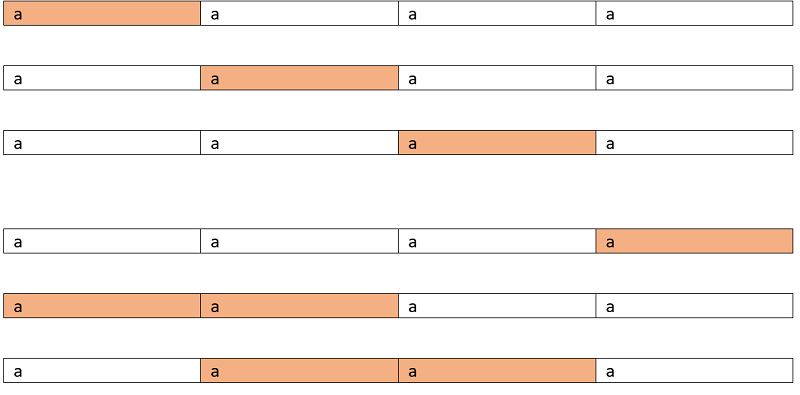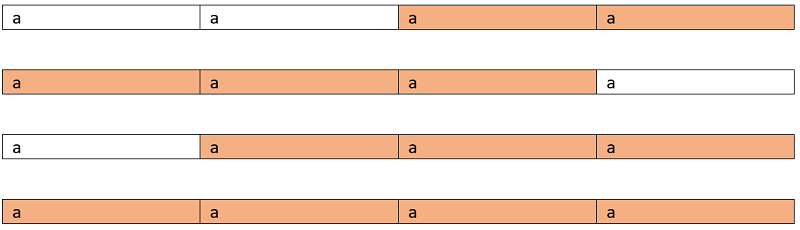Q:

# Longest Palindromic Substring

Given a string str, find the longest palindromic substring. A substring need to be consecutive such that for any xixj i<j must be valid in the parent string too. Like "incl" is a substring of "includehelp" while "iel" is not

Input:

The first line of input contains an integer T, denoting the no of test cases then T test cases follow. Each test case contains a tring str.

Output:

For each test case output will be a string which is the longest palindromic substring could be formed from the string str. There can be many valid answers, all are correct.

Constraints:

```1 <= T <= 100
1 <= length of string str <= 300
```

Example:

```Input:
test case:2

First test case:
Input string:
"aaaa"

Output:
Longest palindromic substring is: "aaaa"

Second test case:
Input string:
"abcaba"

Output:
Total count of palindromic sub-sequence is: "aba"
```

Explanation:

Test case 1: Input: "aaaa"

The valid palindromic substrings are shown below:

Marked cells are character taken in subsequence:So the longest one is "aaaa"

For the second test case,

```The substrings can be,
"a"
"b"
"c"
"aba"

So the longest one is "aba"```

This can be solved by using DP top-down approach,

1. Initialize a Boolean 2D array dp[n][n] to store whether the substrings are palindrome or not.
2. Initialize max to store max length of substring and p to store the substring itself.
Initially, p is the first character as that's the smallest palindrome of length 1 and max = 1.
3. Fill up the base case,
Base case is that each single character is a palindrome itself. And for length of two, i.e, if adjacent characters are found to be equal then dp[i][i+1]=true, else if characters are different then dp[i][i+1]=false
To understand this lets think of a string like "acaa"
Here dp=false
Whereas for dp =true
```for i=0 to n
// for single length characters
dp[i][i]=true
if(i==n-1)
break;
if(s[i]==s[i+1])
dp[i][i+1]=true
else
dp[i][i+1]=false;
end for
```
4. Compute for higher lengths,
```for len=3 to n
for start=0 to n-len
int end=start+len-1;
// start and end is matching and rest of
if(s[end]==s[start]   && dp[start+1][end-1])
dp[start][end]=true;
Update max=len;
Update p=s.substr(start,len);
else
dp[start][end]=false;
end if
end for
end for
```
5. Final result is stored in p;

So for higher lengths, if starting and ending index is the same then we recur for the remaining characters since we have the sub-problem result stored so we computed that. That's why we just update max to len as we are checking for increasing length at each iteration.

For proper understanding, you can compute the table by hand for the string "abcaba" to understand how it's working.

C++ Implementation

``````#include <bits/stdc++.h>
using namespace std;

string longestPalindrome(string s)
{

int n = s.length();

if (n == 0)
return "";
if (n == 1)
return s;

bool dp[n][n];

string p = string(1, s);
int max = 1;

for (int i = 0; i < n; i++) {
dp[i][i] = true;
if (i < n - 1 && s[i] == s[i + 1]) {
dp[i][i + 1] = true;
if (2 > max) {
p = s.substr(i, 2);
max = 2;
}
}
else if (i < n - 1) {
dp[i][i + 1] = false;
}
}

for (int len = 3; len <= n; len++) {
for (int j = 0; j <= n - len; j++) {
int end = j + len - 1;
if (s[j] == s[end]) {

dp[j][end] = dp[j + 1][end - 1];
if (dp[j][end] && len > max) {
max = len;
p = s.substr(j, len);
}
}
else
dp[j][end] = false;
}
}
return p;
}

int main()
{
int t;

cout << "Enter number of testcases\n";
cin >> t;

while (t--) {
string str;

cout << "Enter the string\n";
cin >> str;

cout << "Longest palindromic substring: " << longestPalindrome(str) << endl;
}

return 0;
}
``````

Output:

```Enter number of testcases
2
Enter the string
anccnakj
Longest palindromic substring: anccna
Enter the string
abcaba
Longest palindromic substring: aba```